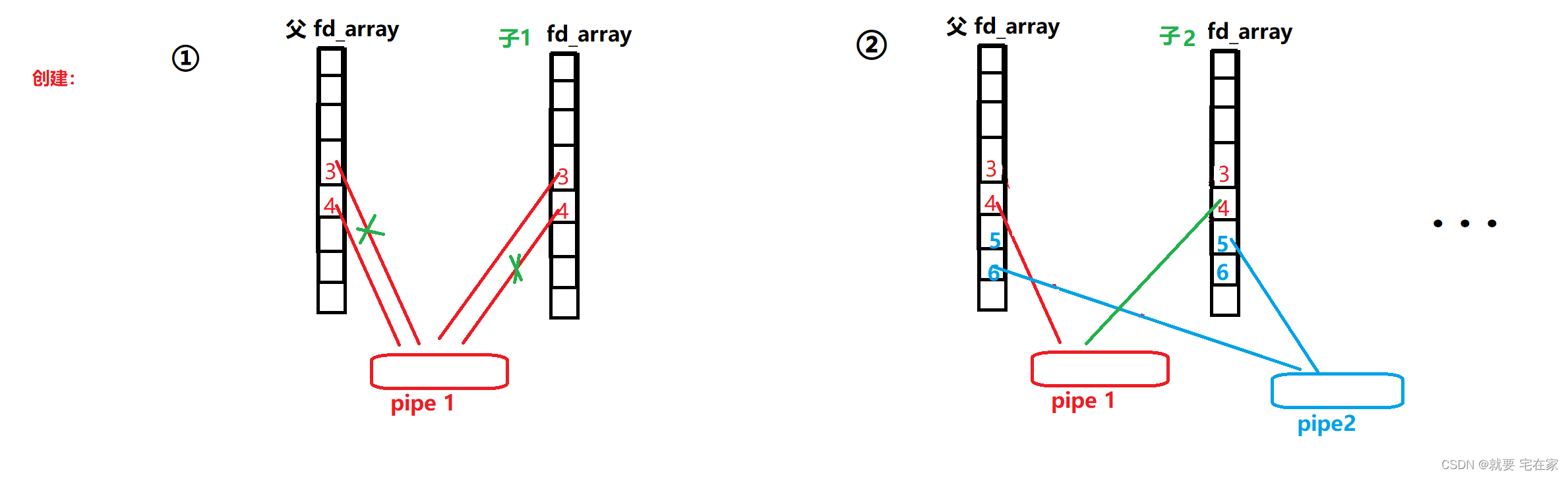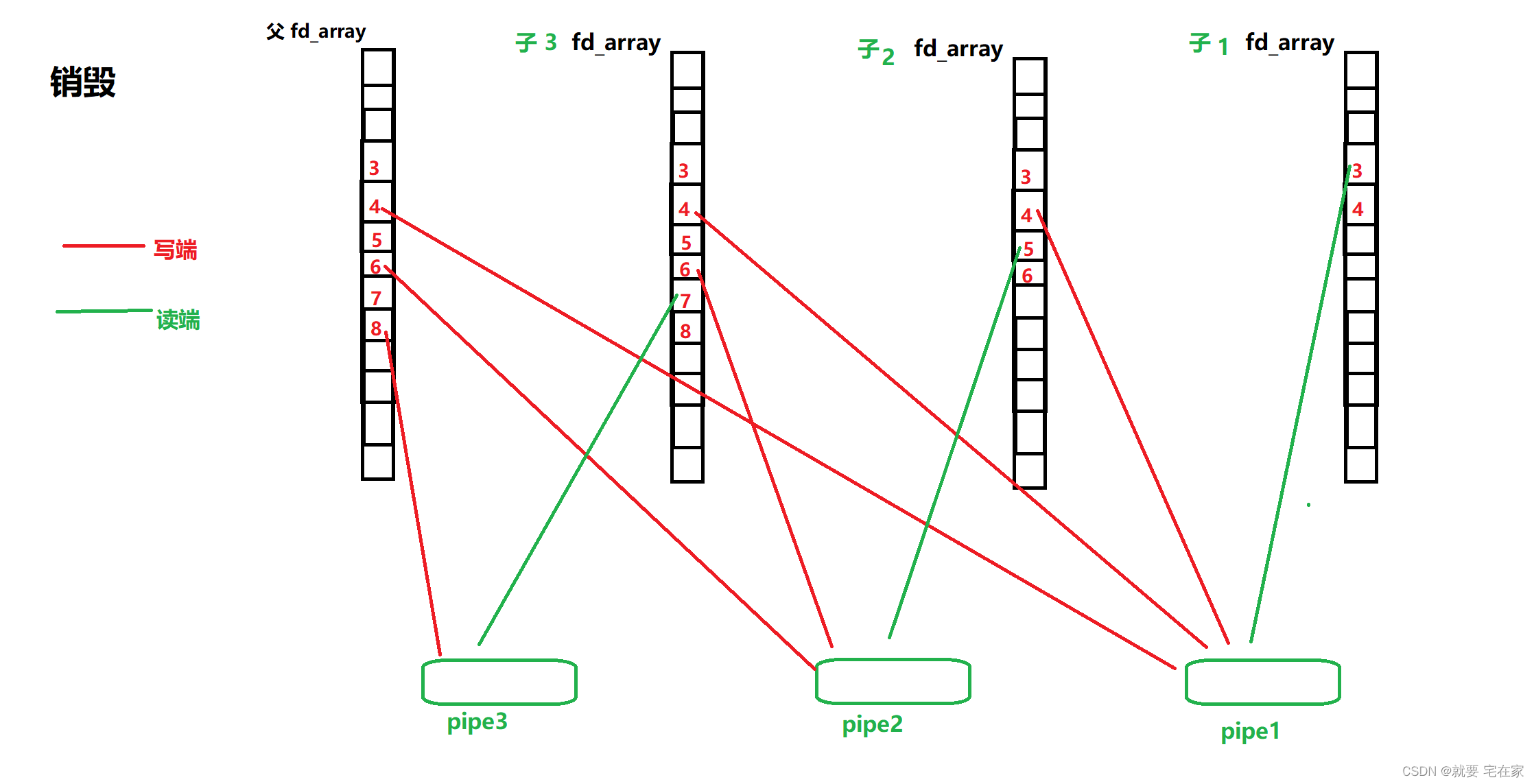一.什么是匿名管道

（一）.pipe原理

（二）.pipe使用

（一）.什么是命名管道

（二）.与匿名管道的联系和区别

（一）.系统指令

（二）.mkfifo

（一）.概念与原理

（二）.代码原理与分析

（三）.进程池管道陷阱

# 二.如何使用匿名管道

## （一）.pipe原理

linux为我们提供了系统接口pipe，用于创建管道进行通信。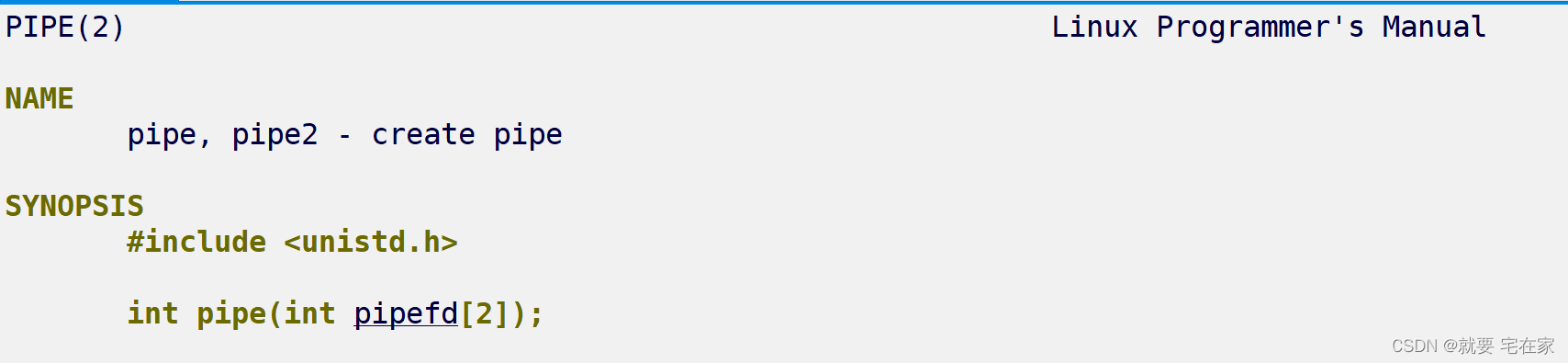参数是长度为2的整形数组，pipefd代表读端文件描述符，pipefd代表写端文件描述符

pipe的使用原理上，就是首先父进程创建一个管道文件，但同时赋予管道文件两个文件描述符。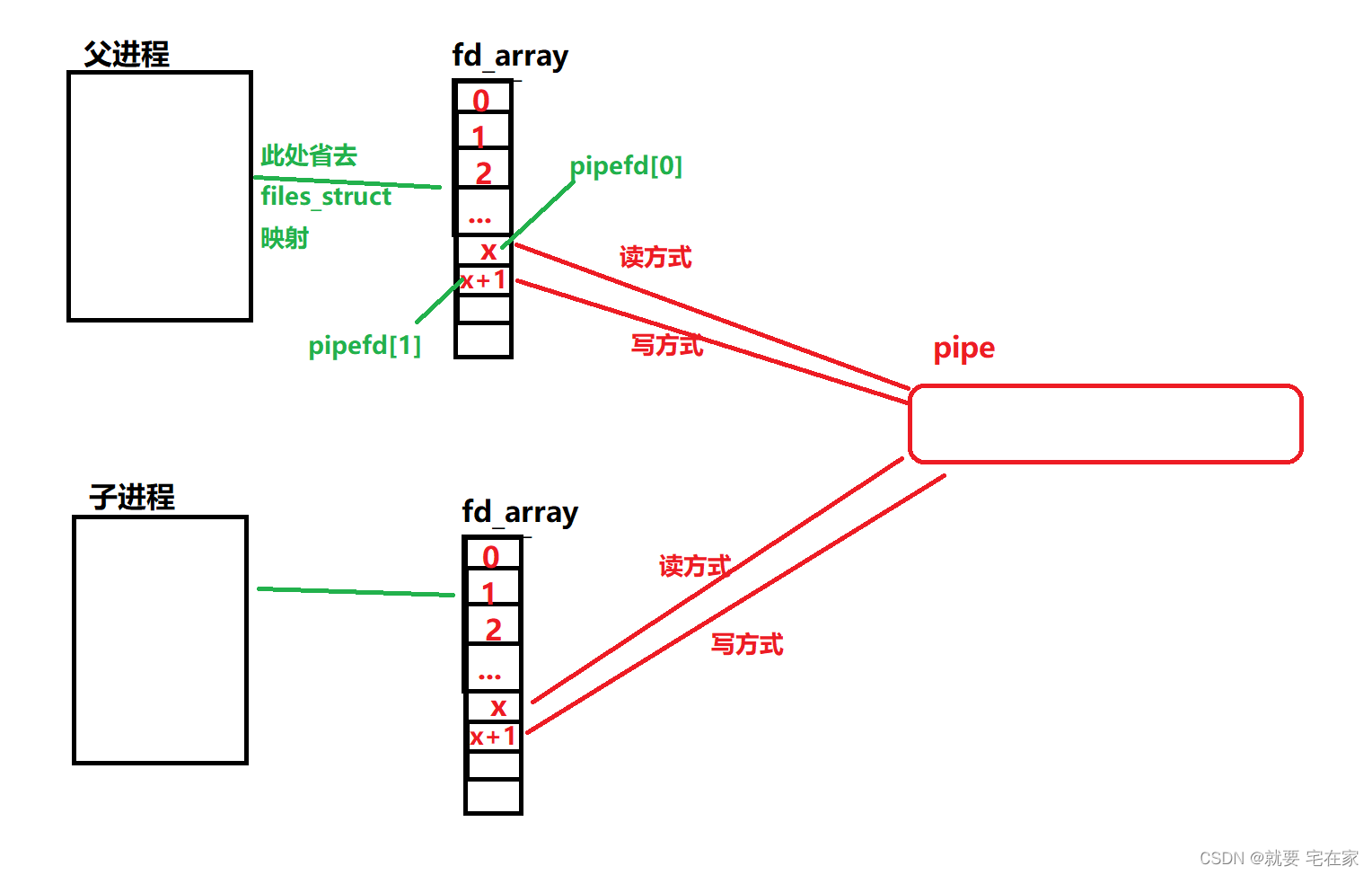## （二）.pipe使用

``````#include<iostream>
#include<cstdio>
#include<unistd.h>
#include<string>
#include<assert.h>
using namespace std;
int main()
{
int pfd = { 0 };
int ret = pipe(pfd);
assert(ret == 0);
pid_t id = fork();
assert(id >= 0);
if(id == 0)
{
close(pfd);//关闭写端
char GetStr = { 0 };
ssize_t i = read(pfd, GetStr, sizeof GetStr);//接收数据
GetStr[i] = '\0';
cout << GetStr << endl;
exit(0);
}
//父进程
close(pfd);
char str = "hello world";
write(pfd, str, sizeof str);//发送数据

return 0;
}``````# 四.命名管道的使用

## （一）.系统指令

演示：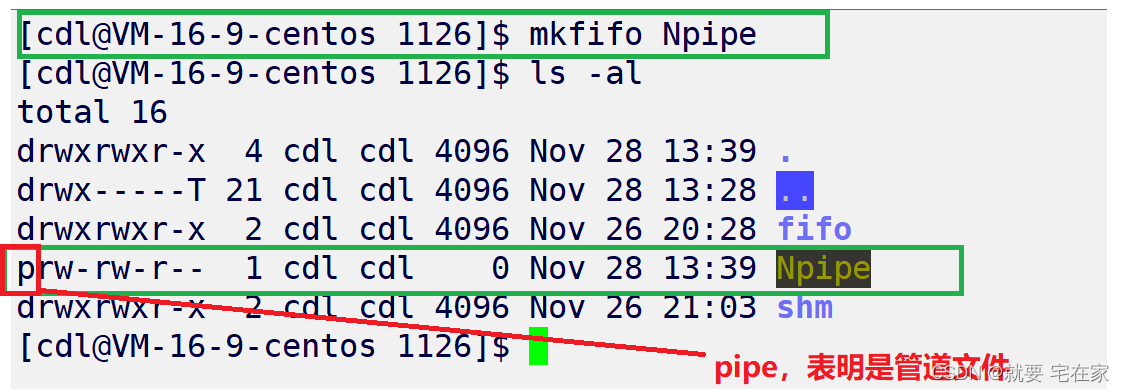## （二）.mkfifo

``````//读端
int i = mkfifo("./fifo.ipc", 0666);//创建命名管道
assert(i >= 0);
int fd = open("./fifo.ipc", O_RDONLY);//打开管道读端，O_RDONLY：只读方式打开
. . .
char buf = { '\0' };
ssize_t s  = read(fd, buf, sizeof buf);//读取数据
. . .
close(fd);//关闭读端管道

//写端
int fd = open("./fifo.ipc", O_WRONLY);打开管道写端，O_WRONLY：只写方式打开
. . .
std::string buf;
std::getline(std::cin, buf);
ssize_t s  = write(fd, buf.c_str(), buf.size());//向管道写入数据
. . .
close(fd);//关闭写端管道
``````

# 五.进程池

## （二）.代码原理与分析

``````//"Command.h"
#pragma once
#include<iostream>
#include<cstdio>
#include<vector>
#include<functional>
using namespace std;
typedef function<void()> func;
vector<func> callCommand;
void Running()
{
cout << "Running now" << endl;
}
void Writing()
{
cout << "Writing now" << endl;
}
void Eating()
{
cout << "Eating now" << endl;
}
void Sleeping()
{
cout << "Sleeping now" << endl;
}
void Testing()
{
cout << "Testing now" << endl;
}

void CommandInit()
{
callCommand.push_back(Running);
callCommand.push_back(Writing);
callCommand.push_back(Eating);
callCommand.push_back(Sleeping);
callCommand.push_back(Testing);
}
void ShowAllCommand()
{
//... if you want you can write one ^-^
}
``````
``````#include<cstdlib>
#include<iostream>
#include<cstdio>
#include<vector>
#include<unistd.h>
#include<sys/wait.h>
#include<sys/fcntl.h>
#include<assert.h>
#include"Command.h"
using namespace std;
#define PROCESS_NUM 5 //number of process is 5

int main()
{
CommandInit(); //init for Command function

vector<pair<pid_t, int>> KvPidFd; //record children process pid & read file fd

for(int i = 0; i < PROCESS_NUM; i++) //creat child process
{
int pipefd = { 0 };
int k = pipe(pipefd);
assert(k == 0); //-1 : creat false
pid_t id = fork();

if(id == 0) //child
{
close(pipefd);
while(1)
{
uint32_t accept = -1;  //uint32_t : unsigned int in 32bit
cout << "*********" << getpid() << endl;

int s = read(pipefd, &accept, sizeof accept);
if(s == 0) break; //if s is zero, that's mean child read zero word in pipe

assert(accept >= 0);
cout << "I am child " << getpid() << " now accept command : ";
callCommand[accept]();  //invoke function
}

cout << "child " << getpid() << "finished work !" << endl;
close(pipefd);
exit(0);
}

//only father process can go to this step
//take chiid pid & it's write fd as a mapping
close(pipefd);
KvPidFd.push_back(make_pair(id, pipefd));
}

srand((unsigned int)time(nullptr) * getpid() * 131);
uint32_t command = 0;
int proc = -1;
int count = 0;

while(1) //father
{
sleep(1);
//random distribute function which will be commanded
command = rand() % callCommand.size();
assert(command >= 0);
proc = rand() % PROCESS_NUM; //random distribute process which will be used
assert(proc >= 0);
write(KvPidFd[proc].second, &command, sizeof(command));
count++;
if(count == 5) break;//stop this loop when calling child five times
}

//close pipe files & revoke children
for(auto kv : KvPidFd)
{
close(kv.second);
}

for(auto kv : KvPidFd)
{
waitpid(kv.first, nullptr, 0);
}

return 0;
}``````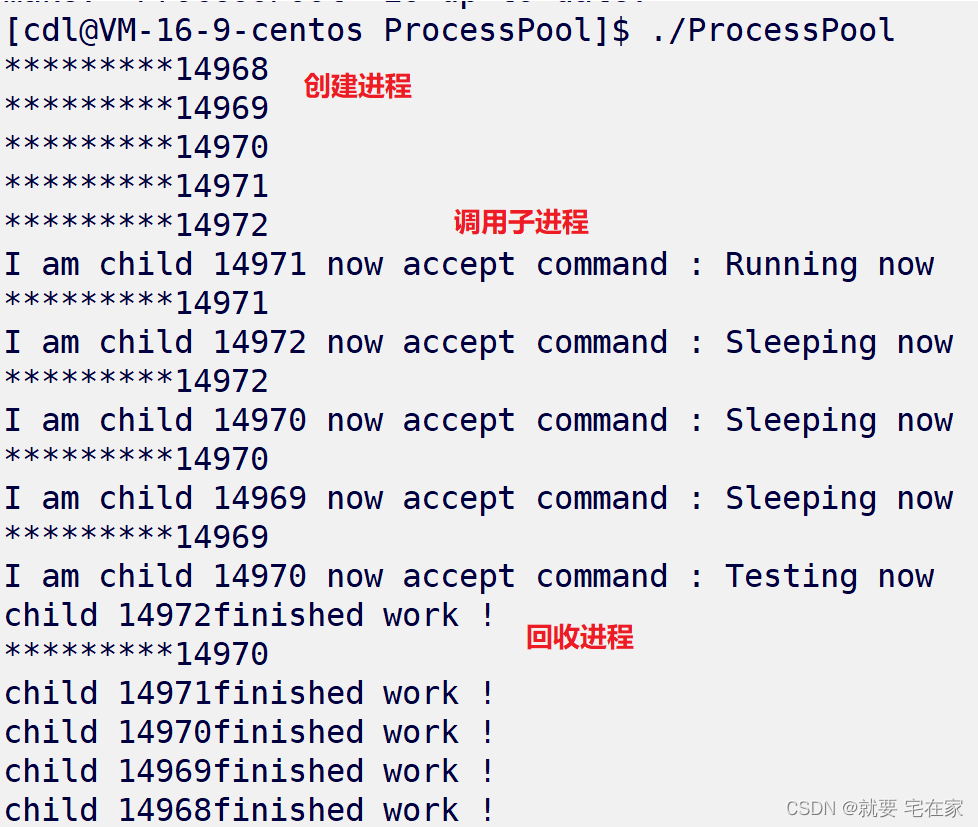## （三）.进程池管道陷阱

有人可能有疑惑，这里有什么陷阱呢？

``````//close pipe files & revoke children
for(auto kv : KvPidFd)
{
close(kv.second);
pid_t id = waitpid(kv.first, nullptr, 0);
assert(id > 0);
cout << "From father : child " << id << "finish work" << endl;
}``````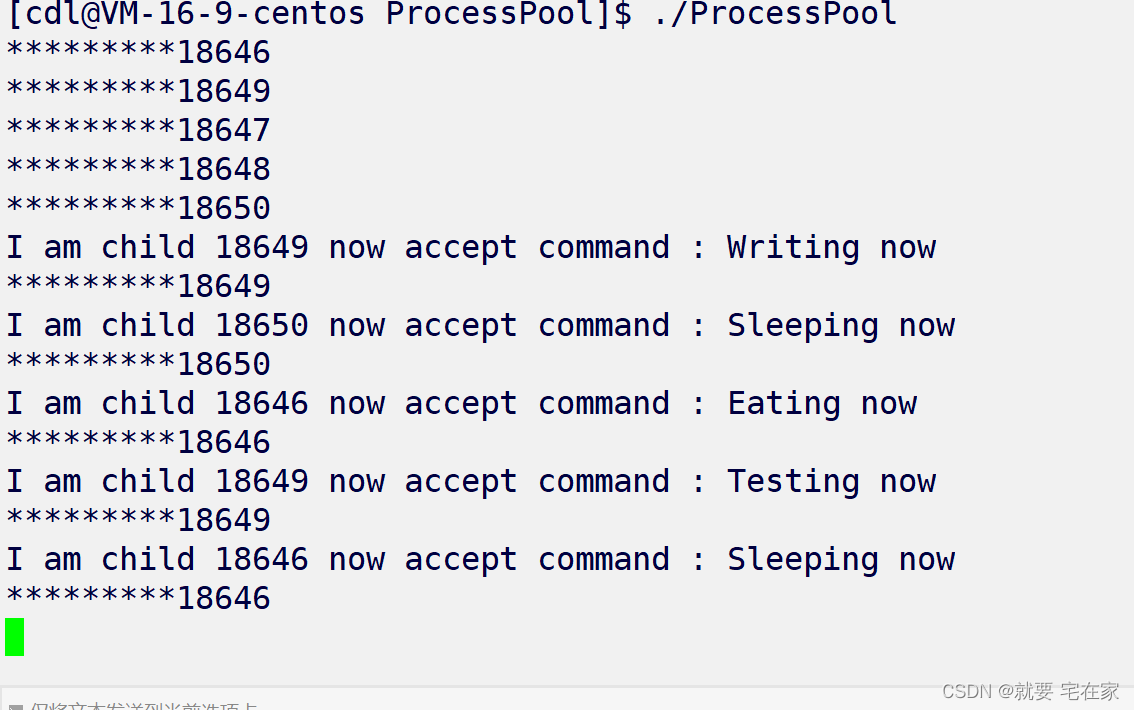不要着急，我们来仔细梳理一下父进程与子进程的管道关系就能得到答案。